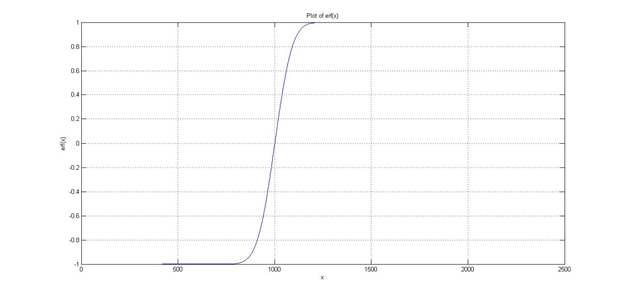While brushing up on some study materials in Mathematics, a familiar function piqued my interest. It was the error function, the solution to the non-elementary integral exp(-x^2) and whose complement is used in determining the conditional probability of bit error due to noise:Or quite simply, the probability of error due to noise.But the real point of interest was the nature of the curve of the function shown below.Now, why be so interested in such a function? When I compared erf(x) with the sigmoid function commonly used in defining the decision boundary in machine learning algorithms, it returned a steeper slope. Then the thought came to me. What would be the differences of using the error function instead of the sigmoid function? Would the cost improve? Would the training accuracy improve?

And so my curiosity got the better of me and I played around with both of the functions to see what would happen.

### Sigmoid vs. Error

First of all, replacing the sigmoid function with the error function outright won’t work. The levels are all wrong. To get both functions to be at similar levels (logistic right?), I add offset to the error function by 1 unit and scale it down by a factor of 2.To mathematically check its similarity to the sigmoid function, I take the correlation of the 2 functions. I am expecting the correlation to be close to 1.

>>x=[-10:0.01:10];

>>y=1./(1+exp(-x)); %the sigmoid function

>>corr(a’,y’) = 0.9901 %correlation is indeed close to 1 which proves the similarity between the 2 functions, this is Pearson’s linear correlation coefficient

>>corr(a’,y’,’type’,’Kendall’) = 0.9565 %Kendall’s tau

>>corr(a’,y’,’type’,’Spearman’) = 0.9912 %Spearman’s rho

To compare both functions visually, I overlay the plots of both functions on the figure below.The eye can easily judge that the rising slope of the error function is steeper than the sigmoid function.

### Testing the performance of the sigmoid and error functions in logistic regression

In order to see the effect of using the error function (a function with a steeper slope) instead of the sigmoid function as a hypothesis in logistic regression, I will be using a 100 sample training set whose final theta will be determined by the fminunc function.The cost at initial values of theta (i.e. 0) are the same for both the sigmoid and error functions, that is 0.693147. However there is a slight difference between the costs of the 2 functions at the final value of theta. Fminunc determined a cost of 0.203506 for the sigmoid function while a cost of 0.201282 was determined for the error function. I am not sure if this is due to the iteration being terminated earlier for the sigmoid function but the diff. is too small to significantly impact our 100 sample training set.

Finally, for a 100 sample training set, both functions arrived at the same train accuracy of 89 after comparing the predictions. I am a bit skeptical though, perhaps the train accuracy of the error function would be higher if samples chanced on the area to the right of the sigmoid boundary but to the left of the error function boundary. A recommended study of this would be how the performance would change with variable sizes of the training set.